Search IntMath
Close

450+ Math Lessons written by Math Professors and Teachers

5 Million+ Students Helped Each Year

1200+ Articles Written by Math Educators and Enthusiasts

Simplifying and Teaching Math for Over 23 Years

# 6. Integration: Inverse Trigonometric Forms

by M. Bourne

Using our knowledge of the derivatives of inverse trigonometric identities that we learned earlier and by reversing those differentiation processes, we can obtain the following integrals, where u is a function of x, that is, u=f(x).

int(du)/sqrt(a^2-u^2)=sin^(-1)(u/a)+K

int(du)/(a^2+u^2)=1/atan^(-1)(u/a)+K

NOTE: Your calculator has sin^(-1) and tan^(-1) buttons, but these create quite a bit of confusion because they are inverse functions, not reciprocals. We could also (better) write these formulas using arcsin and arctan as follows:

int(du)/sqrt(a^2-u^2)=arcsin u/a+K

int(du)/(a^2+u^2)=1/a arctan u/a+K

### Example 1

Integrate: int(dx)/sqrt(49-x^2

Applying the formula, we have:

int(dx)/(sqrt(49-x^2))=int(dx)/(sqrt((7)^2-x^2))

=sin^-1(x/7)+K

We could also write this answer as:

arcsin (x/7)+K

This is the graph of the function we just integrated.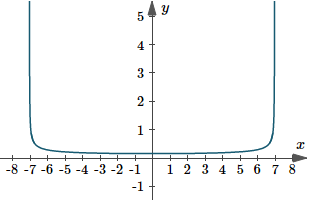Graph of y(x)=1/sqrt(49-x^2).

The next graph is a typical solution graph for the integral we just found, with K=0.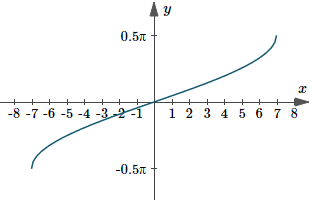Graph of y(x)=arcsin(x/7).

### Example 2

Integrate: int_0^1(2\ dx)/(sqrt(9-4x^2

int_0^1(2 dx)/(sqrt(9-4x^2

Let u=2x then du=2\ dx.

Our integral becomes:

int_0^1(2 dx)/(sqrt(9-4x^2))=int_(x=0)^(x=1)(du)/(sqrt((3)^2-u^2))

=[sin^-1(u/3)]_(x=0)^(x=1)

=[sin^-1 ((2x)/3)]_0^1

=[sin^-1 (2/3)-sin^-1 0]

=0.7297

We could have done it in much fewer steps by leaving it in terms of "u", as follows:

int_0^1(2 dx)/(sqrt(9-4x^2))=int_0^2(du)/(sqrt((3)^2-u^2))

=[sin^-1 (2/3)-sin^-1 0]

=0.7297

Note the change in the limits when dx is changed to du during the integration.

This is because we let u = 2x.

Hence the limits for x of

x = 0 and x = 1

have to be changed for u to

u = 0 and u = 2.

Here is the graph of the integral we just found. It represents the area under the curve y(x)=2/sqrt(9-4x^2) from 0 < x < 1.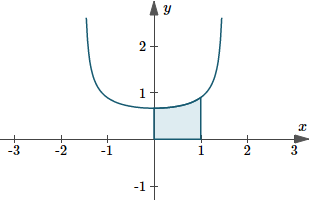Graph of y(x)=2/sqrt(9-4x^2).

### Example 3

Find the area bounded by the curve y=1/(1+x^2) and the lines x = 0, y = 0 and x = 2.

The curve y=1/(1+x^2) lies entirely above the x-axis for all values of x, so to find the area we can simply integrate. (If part of the curve was below the x-axis, we would need to split it into different portions and take absolute values.)

"Area"=int_0^2(dx)/(1+x^2)

=[tan^-1x]_0^2

=[tan^-1 2-tan^-1 0]

=1.1071\ "units"^2

This is the area we found just now.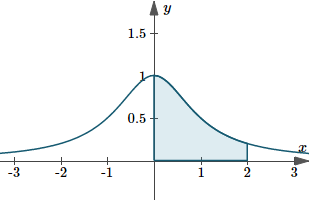Graph of y(x)=1/(1+x^2) showing area between 0 < x < 2.

### Caution

There are a number of integrals of forms which look very similar to the above formulas but are actually different, e.g.

int(dx)/(sqrt(x^2-1)),\ \ int(dx)/(sqrt(1+x^2)), \ \ int(dx)/(1-x^2),\ "etc."

We will develop methods to solve these in a later section. (See Integration by Trigonometric Substitution.)

## Exercises

Integrate each of the given functions:

1. int(3\ dx)/(25+16x^2)

We can write our integral as:

int(3\ dx)/(25+16x^2)=3int(dx)/((5)^2+(4x)^2

For the formula, we need:

a = 5; u = 4x; du = 4\ dx.

Re-arranging that last expression gives: (du)/4=dx.

We are now ready to perform the integration.

int(3\ dx)/(25+16x^2)=3/4int(du)/((5)^2+u^2)

=3/4xx1/5tan^-1 (u/5)+K

=3/20tan^-1 ((4x)/5)+K

We could also write this answer as:

3/20 arctan (4x)/5+K

2. int(2\ dx)/(x^2+8x+17)

int(2 dx)/(x^2+8x+17

Now

x^2+8x+17=(x^2+8x+16)+1

=(x+4)^2+1

So if we let u = x + 4, then du = dx and we have:

int(2\ dx)/(x^2+8x+17)=2int(du)/(u^2+1)

=2\ tan^-1u+K

=2\ tan^-1(x+4)+K

Of course, we could also write this answer as

2\ arctan(x+4)+K

3. int(dx)/(sqrt(2x-x^2)

int(dx)/(sqrt(2x-x^2)

This is not in the form of either of our new formulas, but we can do some juggling to get it into a useful form.

First, we recognise that

2x-x^2=-(x^2-2x)

We now add 1 at the front, then compensate for it inside the bracket:

=1-(x^2-2x+1)

=1-(x-1)^2

With a = 1; u = x - 1, and du = dx, our integral becomes:

int(dx)/(sqrt(2x-x^2))=int(du)/(sqrt(1-u^2))

=arcsin u+K

=arcsin(x-1)+K

4. Find the area bounded by the curve ysqrt(4-x^2)=1 and the lines x = 0, y = 0 and x = 1.

The curve's function can be written:

y=1/sqrt(4-x^2)

This curve is completely above the x-axis for all values of x such that -2 < x < 2, as we can see in the graph below. (It's not defined for any other values of x).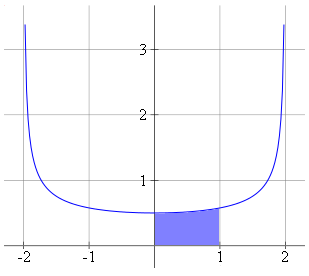So to find the required area (the shaded portion) we can simply integrate.

A=int_0^1 1/(sqrt(4-x^2))dx

=[arcsin(x/2)]_0^1

=pi/6-0

=0.5236

## Problem SolverThis tool combines the power of mathematical computation engine that excels at solving mathematical formulas with the power of GPT large language models to parse and generate natural language. This creates math problem solver thats more accurate than ChatGPT, more flexible than a calculator, and faster answers than a human tutor. Learn More.